# Euler's formula facts for kids

Kids Encyclopedia Facts

In complex analysis, Euler's formula, also sometimes called Euler's relation, is an equation involving complex numbers and trigonometric functions. More specifically, it states that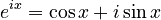$e^{ix} = \cos x + i \sin x$

where x is a real number, e is Euler's number and i is the imaginary unit.

It makes a relation between trigonometric functions and exponential functions of complex numbers. It is named after Leonhard Euler, who published it in 1748. When he published it, Euler said that the angle must be a real number. Later, it turned out that the formula also works if the angle is not a real number, but a complex one.

When the angle is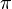$\pi$ and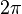$2\pi$, Euler's formula becomes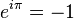$e^{i\pi} = -1$ and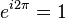$e^{i2\pi} = 1$, respectively.

## Images for kidsEuler's formula Facts for Kids. Kiddle Encyclopedia.